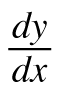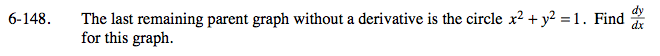### Home > CALC > Chapter 6 > Lesson 6.4.2 > Problem6-148

6-148.

The last remaining parent graph without a derivative is the circle x2 + y2 = 1. Findfor this graph. Homework Help ✎Recall that a circle is NOT a function.

Before differentiating, you could solve for y and get

Then find the derivative of each piece separately, or.....

You could use implicit differentiation!

Implicit differentiation:

$\frac{d}{dx}(x^{2}+y^{2})=\frac{d}{dx}(1)$